# To graph : The quadratic equation y = 10 + 25 x − x 3 in a graphing window.### Precalculus: Mathematics for Calcu...

6th Edition
Stewart + 5 others
Publisher: Cengage Learning
ISBN: 9780840068071### Precalculus: Mathematics for Calcu...

6th Edition
Stewart + 5 others
Publisher: Cengage Learning
ISBN: 9780840068071

#### Solutions

Chapter 1.9, Problem 9E
To determine

## To graph: The quadratic equation y=10+25x−x3 in a graphing window.

Expert Solution

### Explanation of Solution

Given information:

Graph:

The graph of the quadratic equation y=10+25xx3 can be sketched in the cartesian plane,

Consider the quadratic equation, y=10+25xx3 .

Rewrite the given equation:

y=10+25xx3y=x3+25x+10y=x(25x2)+10 ,

Now put the values of x in the equation to find the values of y or to find the real roots of y with the help of x .

Substitute the value of x=3 in equation y=x(25x2)+10 :

y=x(25x2)+10y=3(25(3)2)+10y=3(259)+10y=3×16+10

The value of y is:

y=3×16+10y=48+10y=38

Substitute the value of x=2 in equation y=x(25x2)+10 :

y=x(25x2)+10y=2(25(2)2)+10y=2(254)+10y=2×21+10

The value of y is:

y=2×21+10y=42+10y=32

Substitute the value of x=1 in equation y=x(25x2)+10 :

y=x(25x2)+10y=1(25(1)2)+10y=1(251)+10y=1×24+10

The value of y is:

y=1×24+10y=24+10y=14

Substitute the value of x=0 in equation y=x(25x2)+10 :

y=x(25x2)+10y=0(25(0)2)+10y=0(25)+10y=10

Substitute the value of x=1 in equation y=x(25x2)+10 :

y=x(25x2)+10y=1(25(1)2)+10y=1(251)+10y=1×24+10

The value of y is:

y=24+10y=34

Substitute the value of x=2 in equation y=x(25x2)+10 :

y=x(25x2)+10y=2(25(2)2)+10y=2(254)+10y=2×21+10

The value of y is:

y=2×21+10y=42+10y=52

Substitute the value of x=3 in equation y=x(25x2)+10 :

y=x(25x2)+10y=3(25(3)2)+10y=3(253)+10y=3×24+10

The value of y is:

y=3×24+10y=72+10y=82

When the value of x is 3 then value of y is 38 .

Same as the value of x is 2 then value of y is 32 .

The value of x is 1 then value of y is 14 .

The value of x is 0 then value of y is 10 .

The value of x is 1 then value of y is 34 .

The value of x is 2 then value of y is 52 .

The value of x is 3 then value of y is 82 .

Observe that as the value of x increases there is the huge increment in value of y .

Same as the value of x decreases there is a decrement in the value of y .

Steps to plot the graph of the equation y=x3+25x+10 with the help of graphing utility are as follows:

Step 1: Press MODE key.

Step 2: Use the down arrow key to reach FUNC option.

Step 3: Press ENTER key.

Step 4: Press Y= key.

Step 5: Enter the function y=x3+25x+10 .

Step 6: Press WINDOW key. Change the settings to

Xmin=20Xmax=20Ymin=800Ymax=1000

For better view of graph.

Step 8: Press GRAPH key.

The result obtained on the screen is provided below,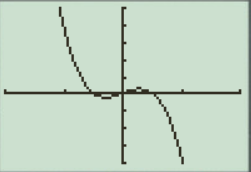Interpretation:

The equation of the function y=x3+25x+10 represents a parabola.

The equation has a wave formed graph.

The x intercepts are the points on xaxis where the graph of the equation touches xaxis .

Recall that the graphical approach to solve the equation simultaneously is observe the point where the graph of equation y=x3+25x+10 intersects the y -axis.

Therefore, the equation y=x3+25x+10 is symmetric about the y axis Initially the graph of the equation y=x3+25x+10 increases when increase the value of x .

a.When the x -axis ranges from [4,4] and y -axis ranges from [4,4] their graph obtained in the screen is provided below.

Steps to plot the graph of the equation y=x3+25x+10 with the help of graphing utility are as follows:

Step 1: Press MODE key.

Step 2: Use the down arrow key to reach FUNC option.

Step 3: Press ENTER key.

Step 4: Press Y= key.

Step 5: Enter the function y=x3+25x+10 .

Step 6: Press WINDOW key. Change the settings to

Xmin=4Xmax=4Ymin=4Ymax=4

For better view of graph.

Step 8: Press GRAPH key.

The result obtained on the screen is provided below,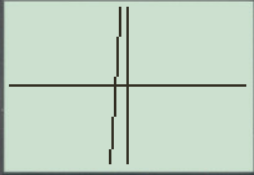This window is not perfect viewing window.

b.

When the x -axis ranges from [10,10] and y -axis ranges from [10,10] their graph obtained in the screen is provided below.

Steps to plot the graph of the equation y=x3+25x+10 with the help of graphing utility are as follows:

Step 1: Press MODE key.

Step 2: Use the down arrow key to reach FUNC option.

Step 3: Press ENTER key.

Step 4: Press Y= key.

Step 5: Enter the function y=x3+25x+10 .

Step 6: Press WINDOW key. Change the settings to

Xmin=10Xmax=10Ymin=10Ymax=10

For better view of graph.

Step 8: Press GRAPH key.

The result obtained on the screen is provided below,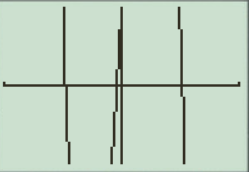This window is not a perfect viewing window.

c.

When the x -axis ranges from [20,20] and y -axis ranges from [100,100] their graph obtained in the screen is provided below.

Steps to plot the graph of the equation y=x3+25x+10 with the help of graphing utility are as follows:

Step 1: Press MODE key.

Step 2: Use the down arrow key to reach FUNC option.

Step 3: Press ENTER key.

Step 4: Press Y= key.

Step 5: Enter the function y=x3+25x+10 .

Step 6: Press WINDOW key. Change the settings to

Xmin=20Xmax=20Ymin=100Ymax=100

For better view of graph.

Step 8: Press GRAPH key.

The result obtained on the screen is provided below,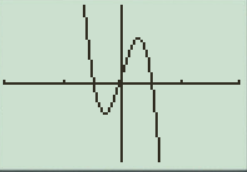This is the perfect viewing window.

d.

When the x -axis ranges from [100,100] and y -axis ranges from [200,200] their graph obtained in the screen is provided below.

Steps to plot the graph of the equation y=x3+25x+10 with the help of graphing utility are as follows:

Step 1: Press MODE key.

Step 2: Use the down arrow key to reach FUNC option.

Step 3: Press ENTER key.

Step 4: Press Y= key.

Step 5: Enter the function y=x3+25x+10 .

Step 6: Press WINDOW key. Change the settings to

Xmin=100Xmax=100Ymin=200Ymax=200

For better view of graph.

Step 8: Press GRAPH key.

The result obtained on the screen is provided below,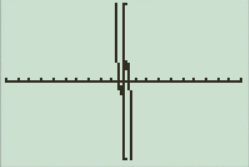This window has large view, in this the graph points are not clear.

So the perfect viewing window of the graph y=x3+25x+10 is option ‘ c[20,20]by[100,100] .

The perfect viewing window is [20,20]by[100,100] , option ‘ c ’.

### Have a homework question?

Subscribe to bartleby learn! Ask subject matter experts 30 homework questions each month. Plus, you’ll have access to millions of step-by-step textbook answers!